# Molecular Calculator Lite

1K+Everyone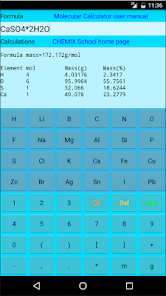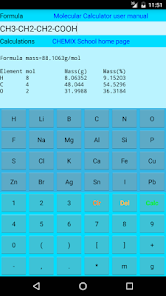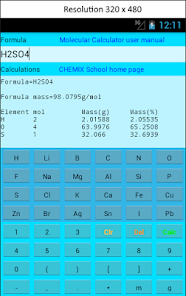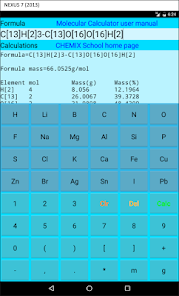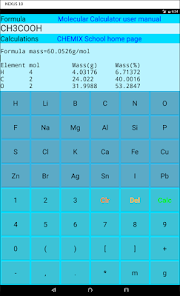Molecular Calculator features:
103 elements and all their stable isotopes.
Easy button-access to 24 of the most common elements. Other elements can be inserted by
using the keyboard - simply touch the formula edit field and keyboard will pop up.
Argumented input:
By inserting 2mNaCl (2 moles of NaCl) it calulates the mass in grams.
By inserting 2gNaCl (2 grams of NaCl) it calculates the mol amount.
By inserting 2mC,CH3COOH (a total of 3 moles of C in the compund) it calculates both moles and mass for the compund.
By inserting 2gC,CH3COOH (a total of 3 grams of C in the compund) it calculates both moles and mass for the compund.
Structural formulas such as CH3-CH2-CH2-COOH can be inserted an calculated.
Crystal water CaCl2*5H2O
Neg. charged ions such as SO4-2 or SO4--
Pos. charged ions such as Fe+3 or Fe+++
Comunds containing isotopes H2O or D2O (Heavy water) or enriched compunds such as CH4
Calculation of statistics such as:
-Moles of different elements or isotopes in a compund.
-Mass of each element in a compund
-Mass% of each element in a compund.
Updated on
Oct 30, 2019

## Data safety